### Values returned by ValueCalc 3.0 screens and add-in functions

To see a full-size image of any of the screens, click on the small "thumbnail" image at the left.

### Cash/Forwards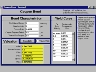Coupon Bond Value; Accrued Interest; Price; Delta; Gamma; Theta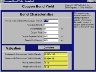Coupon Bond Yield Continuously Compounded and Semiannually Compounded Yields to Maturity; Market and Model Values of Bond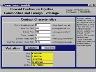Forward Contract Value; Delta; Theta; Rho; Lambda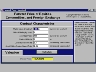Forward Price Delivery Price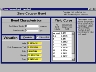Zero-Coupon Bond Value; Continuously Compounded Yield; Delta; Gamma; Theta

### Swaps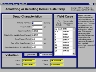Amortizing Swap Value; Delta; Gamma; Theta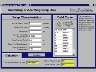Amortizing Swap Rate Equilibrium Fixed Swap Rate; Market and Model Values of Swap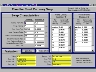Fixed for Fixed Currency Swap Value; Delta and Gamma of Yield in each currency; Delta of each FX; Theta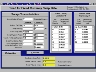Fixed for Fixed Currency Swap Rate Equilibrium Fixed Swap Rate; Market and Model Values of Swap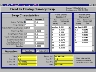Fixed for Floating Currency Swap Value; Delta and Gamma of Yield in each currency; Delta of each FX; Theta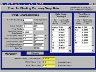Fixed for Floating Currency Swap Rate Equilibrium Fixed Swap Rate; Market and Model Values of Swap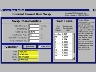Forward Swap Value; Delta; Gamma; Theta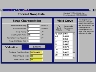Forward Swap Rate Equilibrium Fixed Swap Rate; Market and Model Values of Swap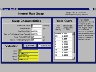Swap Value; Delta; Gamma; Theta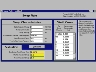Swap Rate Equilibrium Fixed Swap Rate; Market and Model Values of Swap

### Equity/Commodity/FX Options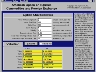American Option Value, Delta, Gamma, Theta, Vega, Rho, and Lambda computed for both Put and Call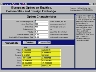European Option Value, Delta, Gamma, Theta, Vega, Rho, and Lambda computed for both Put and Call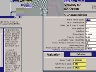Implied Volatility for American Option Implied Volatility; Market and Model Values of Option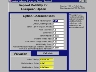Implied Volatility for European Option Implied Volatility; Market and Model Values of Option

### Exotic Options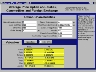Average Price Option Value, Delta, Gamma, Theta, Vega, Rho, and Lambda computed for both Put and Call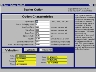Barrier Option Value; Delta; Gamma; Theta; Vega; Rho; Lambda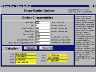Binary Barrier Option Value; Delta; Gamma; Theta; Vega; Rho; Lambda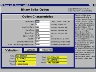Binary Strike Option Value; Delta; Gamma; Theta; Vega; Rho; Lambda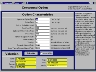Compound Option Value; Delta; Gamma; Theta; Vega; Rho; Lambda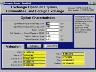Exchange Option Value; Delta and Gamma of spot market value of each asset exchanged; Theta; Vega; Rho; Lambda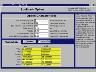Lookback Option Value, Delta, Gamma, Theta, Vega, Rho, and Lambda computed for both Put and Call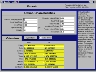Quanto Option Value; Delta; Gamma; Theta; Vega; Rho; Lambda; Vega FX; Lambda FX

### Interest Rate Options: Analytical Valuation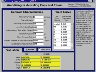Amortizing Caps and Floors Value, Delta, Gamma, Theta, and Vega computed for both Cap and Floor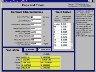Caps and Floors Value, Delta, Gamma, Theta, and Vega computed for both Cap and Floor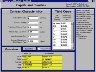Caplets and Floorlets Value, Delta, Gamma, Theta, and Vega computed for both Caplet and Floorlet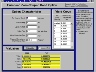European Zero-Coupon Bond Option Value, Delta, Gamma, Theta, and Vega computed for both Put and Call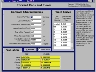Forward Caps and Floors Value, Delta, Gamma, Theta, and Vega computed for both Cap and Floor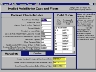Implied Volatility for Caps and Floors Implied Standard Deviation of Short Interest Rate; Market and Model Values of Contract

### Interest Rate Options: Numerical Valuation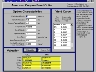American Coupon Bond Option Value, Delta, Gamma, Theta, and Vega computed for both Put and Call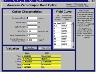American Zero-Coupon Bond Option Value, Delta, Gamma, Theta, and Vega computed for both Put and Call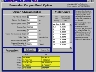Bermudan Coupon Bond Option Value, Delta, Gamma, Theta, and Vega computed for both Put and Call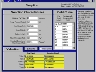Swaption Value, Delta, Gamma, Theta, and Vega computed for both Pay Fixed and Receive Fixed swaptions

webmaster@valuecalc.com